### Tutorial and Application Videos

Learn how Cubis®️ II can support you in your daily workflow with features like an ionizer to eliminate electrostatic charges or electronic signature, audit trail and user management for 21 CFR Part 11 compliance.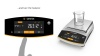Measures the weight of a sample with high accuracy and includes the ability to assign individual sample and lot IDs. Weighing results can be printed and exported manually or automatically.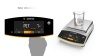Determines the number of identical parts with reference to a known defined weight. The balance will show you the calculated quantity and the total weight.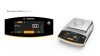Determine the percentage share of an unknown sample in comparison to a reference weight. Weigh the reference weight first, which defines the percentage, then weigh your unknown sample and the application calculates the percentage of this sample compared to the reference.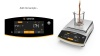Records successive weight values of different components of a recipe as they are added to a vessel, with taring between each component. Records and documents the values of all individual components, as well as the total weight value.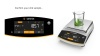Enables you to weigh individual components into different containers and then retrieve the total amount weighed-in.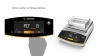Averages multiple individual measurements for accurate weighing of moving samples (for instance live animals) and for weighing in unstable environments. The weighing process can be started manually or automatically.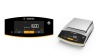Converts a unit of mass using a defined factor. For instance, the application can calculate the weight per unit area, such as gsm? This application will show you the converted weight at the touch of a key.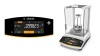Determines the density of solids using the buoyancy method. Weigh the sample first in air and then submerge in liquid, and the application calculates the sample density automatically.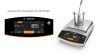Saves a certain number of weight values (components) and statistically analyzes them. Statistical analyses include: Number of components, Average mean weight, Standard deviation, Variation coefficient, Sum of all weights, Lowest weight value (min.), Highest weight value (max.), Difference between min. and max.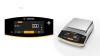Captures and stores the highest positive stable weight value (peak), so that you can read it off for 5 sec after removal of the object.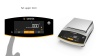Checks if a sample weight is within the specified tolerances, with easy visualization through clear messages in the display. The weighing results can be printed automatically as defined in setup of the application.

The balance displays the weight in the selected weighing unit. In addition you can toggle between 5 different user-defined weighing units.